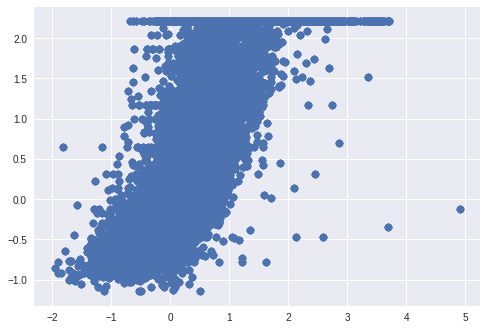# Initial¶

In :
!pip -q install --upgrade --ignore-installed numpy pandas scipy sklearn

In :
!pip -q install catboost
!pip -q install lightgbm

In :
!pip -q install "dask[complete]"

In :
# https://stackoverflow.com/questions/49853303/how-to-install-pydot-graphviz-on-google-colab?rq=1
!pip -q install graphviz
!apt-get install graphviz -qq
!pip -q install pydot

In :
# After this restart your kernel using Ctrl+M+. to reset all loaded libraries to latest ones, that we just installed.


# Import¶

In :
import pandas as pd
import numpy as np
from multiprocessing import Pool
from pandas.io.json import json_normalize
from sklearn.pipeline import make_pipeline, Pipeline
import matplotlib.pyplot as plt
from sklearn.preprocessing import RobustScaler
import queue
import json
import gc
gc.enable()


# Initial 2¶

In :
from sklearn.datasets import fetch_california_housing

In :
dictionary = fetch_california_housing()

Downloading Cal. housing from https://ndownloader.figshare.com/files/5976036 to /root/scikit_learn_data

In :
dictionary.keys()

Out:
dict_keys(['data', 'target', 'feature_names', 'DESCR'])
In :
dictionary.data.shape

Out:
(20640, 8)
In :
xtrain = pd.DataFrame(dictionary.data, index=np.arange(len(dictionary.data)), columns=dictionary.feature_names)
xtrain['Target'] = dictionary.target

In :
new_ = xtrain.iloc[:,:2].to_dict('list')
new_row = []
for i in range(len(xtrain)):
dic_ = {}
for key in new_.keys():
dic_[key] = new_[key][i]
new_row.append(str(dic_))

In :
xtrain['Dict'] = new_row

In :
xtrain.head(2)

Out:
MedInc HouseAge AveRooms AveBedrms Population AveOccup Latitude Longitude Target Dict
0 8.3252 41.0 6.984127 1.02381 322.0 2.555556 37.88 -122.23 4.526 {'MedInc': 8.3252, 'HouseAge': 41.0}
1 8.3014 21.0 6.238137 0.97188 2401.0 2.109842 37.86 -122.22 3.585 {'MedInc': 8.3014, 'HouseAge': 21.0}
In :
for i in range(6):
xtrain = pd.concat([xtrain, xtrain], axis=0, sort=False).reset_index(drop=True)

In :
xtrain.shape

Out:
(1320960, 10)
In :
xtrain.reset_index().to_csv("train.csv", index=False)

In :
del xtrain, dictionary, new_row, new_, dic_
gc.collect()

Out:
143

# Incremental Learning¶

### Data Exploration:¶

In :
!ls

In :
part = pd.read_csv("train.csv", nrows=10)
part.shape

Out:
(10, 11)
In :
columns = part.columns

Out:
index MedInc HouseAge AveRooms AveBedrms Population AveOccup Latitude Longitude Target Dict
0 0 8.3252 41.0 6.984127 1.02381 322.0 2.555556 37.88 -122.23 4.526 {'MedInc': 8.3252, 'HouseAge': 41.0}
1 1 8.3014 21.0 6.238137 0.97188 2401.0 2.109842 37.86 -122.22 3.585 {'MedInc': 8.3014, 'HouseAge': 21.0}
In :
# From above output you will have a basic understanding of type of columns. Divide them so you
# can use same function on similar columns (for exploration etc).
id_columns = ["index"]
num_columns = ["MedInc", "HouseAge", "AveRooms", "AveBedrms", "Population", "AveOccup", "Latitude", "Longitude", "Target"]
obj_columns = []
dict_columns = ["Dict"]
complex_columns = []

In :
index_col = "index"
columns_visited = ["MedInc", "HouseAge", "AveRooms"]

In :
col = "Dict"
df = pd.read_csv("train.csv.zip", usecols = )

df[col] = df[col].map(lambda x: json.loads(x.replace("'", '"')))

column_as_df = json_normalize(df[col])

Out:
HouseAge MedInc
0 41.0 8.3252
1 21.0 8.3014
2 52.0 7.2574
3 52.0 5.6431
4 52.0 3.8462
In :
# Explore this column. Check if you want to keep it or not.
# If you want to scale this column or LableEncode this column,
# do it and maintain a dictionary with all columns as keys and
# corresponding Scalar or LabelEncoder or both as values.

In :
# Calculate Standard Deviation, check if they are constant...
column_as_df.std()

Out:
HouseAge    12.585257
MedInc       1.899776
dtype: float64
In :
column_as_df.plot.kde()

Out:
<matplotlib.axes._subplots.AxesSubplot at 0x7f3699d0fcc0>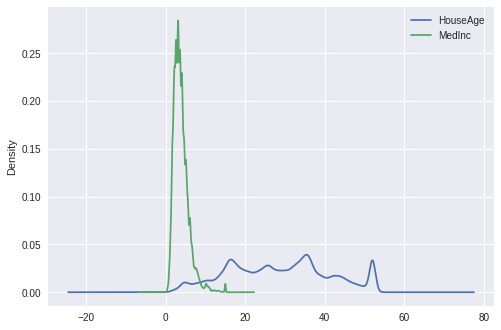In :
columns_selected = ['MedInc', 'HouseAge', 'AveRooms', 'AveBedrms', 'Population', 'AveOccup', 'Latitude', 'Longitude', 'Target']

In :
preprocessing_pipeline = {col: [] for col in columns_selected}

In :
# Here I am doing all at once. But you can do it column by column.
for col_ in columns_selected:
rsc = RobustScaler()
rsc.fit(data[col_].values.reshape(-1, 1)) # You have to do this for RobustScaler...
preprocessing_pipeline[col_].append(rsc)

In :
import pickle
with open("pipeline.pickle", "wb") as fle:
pickle.dump(preprocessing_pipeline, fle)
!ls


Note:

If even one column of dataset is larger than your memory, then you can open that column incrementally (by using chunksize parameter in pd.read_csv), applying some transformation (maybe keeping it in a np.float32 if its a number or maybe keeping only relevant part of a string and throwing all other away.)

But if that column is not fitting into your memory, you can use Dask. (see last section)

### Preprocessing method:¶

In :
# After opening dictionary columns these will change, you can change it to reflect that.
id_columns = []
num_columns = ["MedInc", "HouseAge", "AveRooms", "AveBedrms", "Population", "AveOccup", "Latitude", "Longitude", "Target"]
obj_columns = []
dict_columns = []
complex_columns = []

In :
columns_to_drop_completely = ["index", "Dict"]

In :
# Now that you have a dictionary which has all the columns you need as keys
# and corresponding methods you want to apply to that column sequentially,
# you can make a preprocesing method which will be used to clean data at
# every incremental step.

def preprocess(df):
df.reset_index(drop=True, inplace=True)
df = df.drop(columns_to_drop_completely, axis=1)

# For dict columns:
for col in dict_columns:
if col not in df.columns: pass
col_df.columns = [f"{col}.{subcolumn}" for subcolumn in col_df.columns]
selected_columns = [c for c in dictionary.keys() if c in col_df.columns()]  # Select all columns from this dict-column which we selected before
to_drop = [c for c in col_df.columns if c not in selected_columns]
col_df = col_df.drop(to_drop, axis=1)                                       # Drop all previously unselected sub-columns from this dict_column

df = df.drop([col], axis=1).merge(col_df, right_index=True, left_index=True)

# And so on...

# And then apply all Scalars, LabelEncoders to all columns selected...
for col_ in columns_selected:
# RobustScaler at position 0
rsc = preprocessing_pipeline[col_]
df[col_] = rsc.transform(df[col_].values.reshape(-1, 1)).reshape(1, -1) # Because of RobustScaler

return df


Note:

One thing to notice here is that we fitted methods (like LabelEncoder's, Scalars's etc.) during exploration to whole data column and we will use that to transform data at every incremental step here. Because, in each batch, there might be some data missing and if we had used different LabelEncoder's, Scaler's etc. for each batch, these methods wouldn't have given same result for same category (say). That's why to be on safe side, we already have fitted to whole columns during exploration.

### Method 1: Using Pandas¶

In :
import lightgbm as lgb
import xgboost as xgb
import catboost as cb # CatBoost is currently making its incremental learner: https://github.com/catboost/catboost/issues/464
from sklearn.model_selection import train_test_split

In :
incremental_dataframe = pd.read_csv("train.csv.zip", chunksize=100000) # Number of lines to read.
# This method will return a kind of sequential file reader reading 'chunksize' lines every time.
# To read file from starting again, you will have to call this method again.

In :
lgb_params = {
'objective': 'regression',
'verbosity': 0,
}
# First three are necessary for incremental learning.
xgb_params = {
'update':'refresh',
'process_type': 'update',
'refresh_leaf': True,
'silent': True,
}


For intro on Parallel programming in Python read my post here.

In :
# For saving regressor for next use.
lgb_estimator = None
xgb_estimator = None

for df in incremental_dataframe:
df = preprocess(df)

xtrain, xvalid, ytrain,  yvalid = train_test_split(df.drop(['Target'], axis=1), df['Target'] )

lgb_estimator = lgb.train(lgb_params,
init_model=lgb_estimator, # Pass partially trained model
train_set=lgb.Dataset(xtrain, ytrain),
valid_sets=[lgb.Dataset(xvalid, yvalid)],
valid_names=["Valid"],
early_stopping_rounds = 50,
keep_training_booster=True, # For incremental learning
num_boost_round=70,
verbose_eval=50) # Output after each of 50th times

#xgb_model = xgb.train(xgb_params, dtrain=(xtrain, ytrain),
#                      evals = (xgb.DMatrix(x_tr, y_tr), "Valid"),
#                      xgb_model = xgb_estimator) # Pass partially trained model

del df, xtrain, ytrain, xvalid, yvalid
gc.collect()

Training until validation scores don't improve for 50 rounds.
	Valid's l2: 0.0963618
Did not meet early stopping. Best iteration is:
	Valid's l2: 0.0864367
Training until validation scores don't improve for 50 rounds.
	Valid's l2: 0.0743662
Did not meet early stopping. Best iteration is:
	Valid's l2: 0.0663257
Training until validation scores don't improve for 50 rounds.
	Valid's l2: 0.0630322
	Valid's l2: 0.0560808
Did not meet early stopping. Best iteration is:
	Valid's l2: 0.054903
Training until validation scores don't improve for 50 rounds.
	Valid's l2: 0.0475982
Did not meet early stopping. Best iteration is:
	Valid's l2: 0.0452189
Training until validation scores don't improve for 50 rounds.
	Valid's l2: 0.0434777
	Valid's l2: 0.0399569
Did not meet early stopping. Best iteration is:
	Valid's l2: 0.0399569
Training until validation scores don't improve for 50 rounds.
	Valid's l2: 0.0358432
Did not meet early stopping. Best iteration is:
	Valid's l2: 0.034916
Training until validation scores don't improve for 50 rounds.
	Valid's l2: 0.0324789
Did not meet early stopping. Best iteration is:
	Valid's l2: 0.0307512
Training until validation scores don't improve for 50 rounds.
	Valid's l2: 0.0302787
	Valid's l2: 0.0282038
Did not meet early stopping. Best iteration is:
	Valid's l2: 0.0278374
Training until validation scores don't improve for 50 rounds.
	Valid's l2: 0.025847
Did not meet early stopping. Best iteration is:
	Valid's l2: 0.0249167
Training until validation scores don't improve for 50 rounds.
	Valid's l2: 0.0234998
	Valid's l2: 0.022146
Did not meet early stopping. Best iteration is:
	Valid's l2: 0.022146
Training until validation scores don't improve for 50 rounds.
	Valid's l2: 0.0203596
Did not meet early stopping. Best iteration is:
	Valid's l2: 0.019881
Training until validation scores don't improve for 50 rounds.
	Valid's l2: 0.0188786
Did not meet early stopping. Best iteration is:
	Valid's l2: 0.0180671
Training until validation scores don't improve for 50 rounds.
	Valid's l2: 0.0173369
	Valid's l2: 0.016562
Did not meet early stopping. Best iteration is:
	Valid's l2: 0.016357
Training until validation scores don't improve for 50 rounds.
	Valid's l2: 0.0173281
Early stopping, best iteration is:
	Valid's l2: 0.0170706

In :
test = pd.read_csv("train.csv", nrows = 10000)

In :
preds = lgb_estimator.predict(preprocess(test).drop(['Target'], axis=1))
preds = preprocessing_pipeline['Target'].inverse_transform(preds.reshape(-1, 1)).reshape(1, -1)
true = test['Target']
plt.scatter(preds, true)

Out:
<matplotlib.collections.PathCollection at 0x7f229a7b9ac8>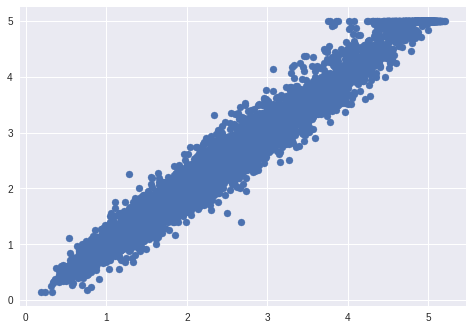Note: You should only use Dask in case of Big Data, where it is not able to fit in your memory.

In :
import dask

In :
import dask.dataframe as dd
client = Client(processes=False, threads_per_worker=4, n_workers=4, memory_limit='8GB')
client

Out:

### Client

• Scheduler: inproc://172.28.0.2/805/1

### Cluster

• Workers: 4
• Cores: 16
• Memory: 32.00 GB
In :
df = dd.read_csv("train.csv", blocksize=25e6)

In :
df.npartitions

Out:
8
In :
df.visualize(size="7,5!")

Out: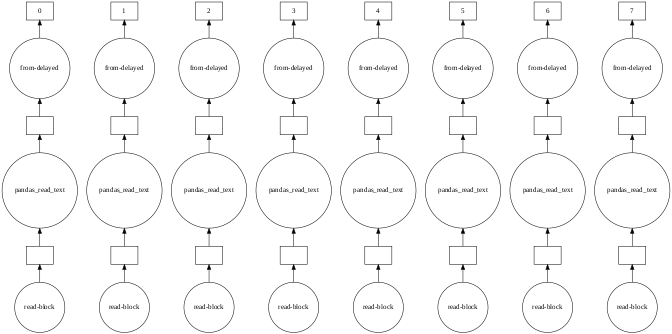In :
df.head()

Out:
index MedInc HouseAge AveRooms AveBedrms Population AveOccup Latitude Longitude Target Dict
0 0 8.3252 41.0 6.984127 1.023810 322.0 2.555556 37.88 -122.23 4.526 {'MedInc': 8.3252, 'HouseAge': 41.0}
1 1 8.3014 21.0 6.238137 0.971880 2401.0 2.109842 37.86 -122.22 3.585 {'MedInc': 8.3014, 'HouseAge': 21.0}
2 2 7.2574 52.0 8.288136 1.073446 496.0 2.802260 37.85 -122.24 3.521 {'MedInc': 7.2574, 'HouseAge': 52.0}
3 3 5.6431 52.0 5.817352 1.073059 558.0 2.547945 37.85 -122.25 3.413 {'MedInc': 5.6431, 'HouseAge': 52.0}
4 4 3.8462 52.0 6.281853 1.081081 565.0 2.181467 37.85 -122.25 3.422 {'MedInc': 3.8462, 'HouseAge': 52.0}

NOTE:

Dask doesn't have equivalent fucntion of pandas's json_normalize. But we can use Dask's to_bag function and bag's capability to handle JSON to our advantage.

This method is taken from a great kernel by Misha Lisovyi, here, on Kaggle.

In :
# Convert string Series to dictionary Series
df['Dict'] = df['Dict'].apply(lambda x: json.loads(x.replace("'", '"')), meta=('Dict', 'f8'))

In :
dict_col_keys = {
'Dict': ['MedInc', 'HouseAge']
}

In :
for dic_col in dict_col_keys:
for key in dict_col_keys[dic_col]:
df[f'{dic_col}.{key}'] = df[dic_col].to_bag().pluck(key).to_dataframe().iloc[:,0]

In :
df.head()

Out:
index MedInc HouseAge AveRooms AveBedrms Population AveOccup Latitude Longitude Target Dict Dict.MedInc Dict.HouseAge
0 0 8.3252 41.0 6.984127 1.023810 322.0 2.555556 37.88 -122.23 4.526 {'MedInc': 8.3252, 'HouseAge': 41.0} 8.3252 41.0
1 1 8.3014 21.0 6.238137 0.971880 2401.0 2.109842 37.86 -122.22 3.585 {'MedInc': 8.3014, 'HouseAge': 21.0} 8.3014 21.0
2 2 7.2574 52.0 8.288136 1.073446 496.0 2.802260 37.85 -122.24 3.521 {'MedInc': 7.2574, 'HouseAge': 52.0} 7.2574 52.0
3 3 5.6431 52.0 5.817352 1.073059 558.0 2.547945 37.85 -122.25 3.413 {'MedInc': 5.6431, 'HouseAge': 52.0} 5.6431 52.0
4 4 3.8462 52.0 6.281853 1.081081 565.0 2.181467 37.85 -122.25 3.422 {'MedInc': 3.8462, 'HouseAge': 52.0} 3.8462 52.0
In :
df.visualize(size="20,10!")

Out: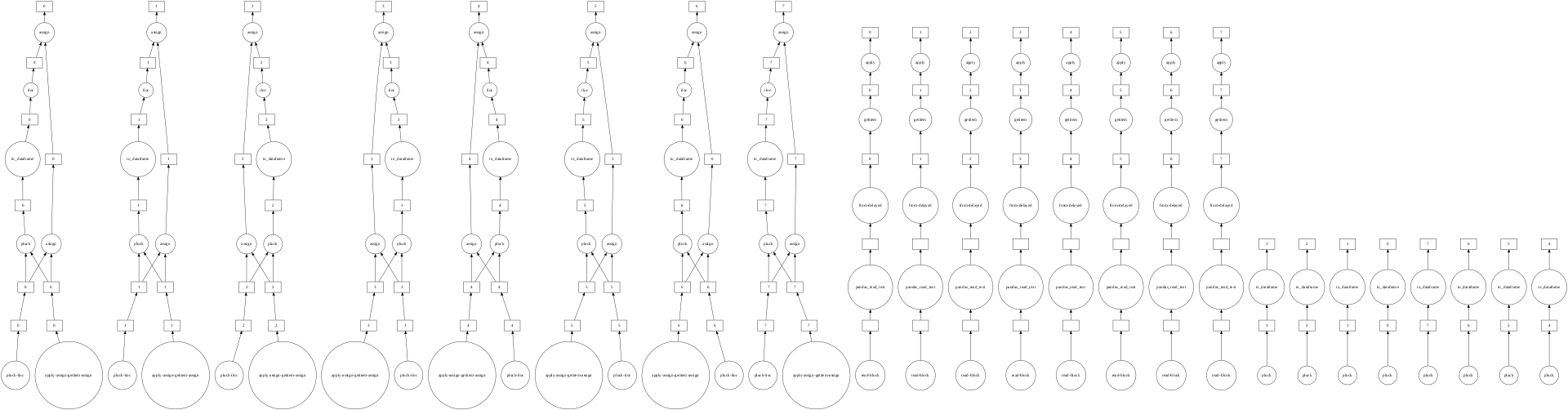In :
columns_to_drop = ['index', 'Dict', 'Dict.MedInc', 'Dict.HouseAge']

In :
df = df.drop(columns_to_drop, axis=1)

In :
df.head()

Out:
MedInc HouseAge AveRooms AveBedrms Population AveOccup Latitude Longitude Target
0 8.3252 41.0 6.984127 1.023810 322.0 2.555556 37.88 -122.23 4.526
1 8.3014 21.0 6.238137 0.971880 2401.0 2.109842 37.86 -122.22 3.585
2 7.2574 52.0 8.288136 1.073446 496.0 2.802260 37.85 -122.24 3.521
3 5.6431 52.0 5.817352 1.073059 558.0 2.547945 37.85 -122.25 3.413
4 3.8462 52.0 6.281853 1.081081 565.0 2.181467 37.85 -122.25 3.422
In :
df.isnull().sum().compute()

Out:
MedInc        0
HouseAge      0
AveRooms      0
AveBedrms     0
Population    0
AveOccup      0
Latitude      0
Longitude     0
Target        0
dtype: int64

You cannot re-input scaled values from your dataframe directly into its columns (It has to be a series). So, we will Scale Array and directly use it to train our model.

In :
# Necessary for converting dataframe to array. Takes specified length from each block.
lengths = []
for part in df.partitions:
l = part.shape.compute()
lengths.append(l)
print(l, part.shape)

182557 9
181760 9
181750 9
181756 9
181750 9
181087 9
180446 9
49854 9

In :
X, y = df.drop(['Target'], axis=1).to_dask_array(lengths=lengths) , df['Target'].to_dask_array(lengths=lengths)

In :
Xo = dask.array.zeros((X.shape,1), chunks=(200000,1))
for i, col_ in enumerate(df.columns):
if col_ == "Target":
rsc = RobustScaler()
y = rsc.fit_transform(y.reshape(-1, 1)).reshape(1, -1)
else:
rsc = RobustScaler()
temp = rsc.fit_transform(X[:,i].reshape(-1, 1))

In :
Xo = Xo[:, 1:]

In :
Xo[-5:].compute()

Out:
array([[-9.11033660e-01, -2.10526316e-01, -1.32135031e-01,
8.99459515e-01, -3.45010616e-01, -3.05382018e-01,
1.32631579e+00, -7.09677419e-01],
[-4.54049344e-01, -5.78947368e-01,  5.33792434e-01,
2.84432167e+00, -8.64118896e-01,  3.53090353e-01,
1.32894737e+00, -7.41935484e-01],
[-8.46968724e-01, -6.31578947e-01, -3.23698645e-02,
7.58319669e-01, -1.73036093e-01, -5.80589452e-01,
1.31315789e+00, -7.44623656e-01],
[-7.70292580e-01, -5.78947368e-01,  4.48869608e-02,
1.31076550e+00, -4.55414013e-01, -8.17678848e-01,
1.31315789e+00, -7.71505376e-01],
[-5.31184078e-01, -6.84210526e-01, -1.72501711e-03,
1.20784296e+00,  2.30360934e-01, -2.39353267e-01,
1.29736842e+00, -7.50000000e-01]])

To make blocks for both of equal size. Otherwise you might get broadcast error.

In :
Xo = Xo.rechunk({1: Xo.shape})
Xo = Xo.rechunk({0: 200000})
y = y.rechunk({0: 200000})

In :
tr_len = 0.8*Xo.shape
xtrain, ytrain = Xo[:tr_len], y[:tr_len]
xvalid, yvalid = Xo[tr_len:], y[tr_len:]
xtrain.shape, ytrain.shape, xvalid.shape, yvalid.shape

Out:
((1056768, 8), (1056768,), (264192, 8), (264192,))
In :
from dask_ml.linear_model import LinearRegression

In :
est = LinearRegression()

In :
est.fit(xtrain, y=ytrain)

Out:
LinearRegression(C=1.0, class_weight=None, dual=False, fit_intercept=True,
intercept_scaling=1.0, max_iter=100, multiclass='ovr', n_jobs=1,
solver_kwargs=None, tol=0.0001, verbose=0, warm_start=False)
In :
preds = est.predict(xvalid)

In :
preds[0:10].compute()

Out:
array([ 0.35169143,  0.46621322,  0.41237802,  0.28285737,  0.24595807,
0.08746062,  0.24150728,  0.26845929, -0.11211374,  0.40954053])
In :
plt.scatter(preds.compute(), yvalid.compute())

Out:
<matplotlib.collections.PathCollection at 0x7fcf807ecb70>